Include Top

# Shewhart ARL

1. Click SigmaXL > Templates & Calculators > Control Chart Templates > Average Run Length (ARL) Calculators> Shewhart ARL. This template is also located at SigmaXL > Control Charts > Control Chart Templates> Average Run Length (ARL) Calculators > Shewhart ARL.
2. The default template settings are Specify = Exact (Test 1 Only), Subgroup Size = 1, Skewness = 0, Kurtosis (Normal is 0) = 0.Notes: Specify Exact (Test 1 Only) or Monte Carlo using the drop-down list. Parameters to be specified will be shown in yellow highlight, otherwise they are hidden. Exact uses the normal or Pearson cumulative distribution function and is fast. Monte Carlo simulation allows you to assess the ARL performance of all 8 Tests for Special Causes. Test 1 - 1 point more than 3 standard deviations from the center line (CL) is always applied. Monte Carlo simulation also produces the table of Run Length Standard Deviation and Percentiles (scroll right to view). Both methods allow you to assess robustness to nonnormality.

All ARL calculations for Shewhart use a standardized in-control mean=0 and sigma=1.

3. Click the Calculate Shewhart ARL button to reproduce the ARL table and chart.As discussed in the introduction, the ARL0 (in-control ARL with 0 shift in mean) for the Shewhart chart is 370.4. The ARL1 for a small 1 sigma shift in mean is 43.89, so is slow to detect. On the other hand, a large 3 sigma shift in mean has an ARL = 2.0, so is detected rapidly.

4. We will now assess ARL for a Shewhart X-bar chart. Select Specify = Exact (Test 1 Only). Enter Subgroup Size = 4, Skewness = 0, Kurtosis (Normal is 0) = 0.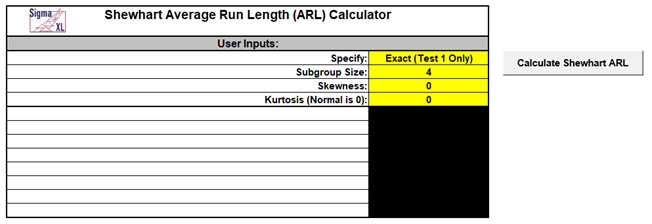5. Click the Calculate Shewhart ARL button to produce the ARL table and chart for these settings: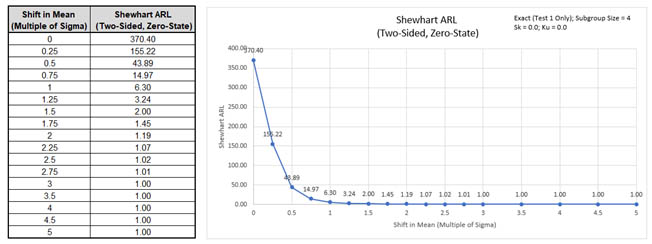The ARL0 for the Shewhart x-bar chart is the same as the Individuals chart, 370.4. The ARL1 for a small 1 sigma shift in mean is 6.3, so is much more rapid to detect than the Individuals ARL1 of 43.89, so if possible, subgrouping should always be used.

Note: The ARL for subgroup averages is adjusted by using the sigma of averages, sigma/√n. For example, with a subgroup size of 4, the ARL1 values at shift in mean = 1 will match the ARL performance of an Individuals chart with shift in mean = 2 sigma.

6. Now we will assess robustness to nonnormality. Enter Specify = Exact (Test 1 Only), Subgroup Size = 1, Skewness = 2, Kurtosis (Normal is 0) = 6.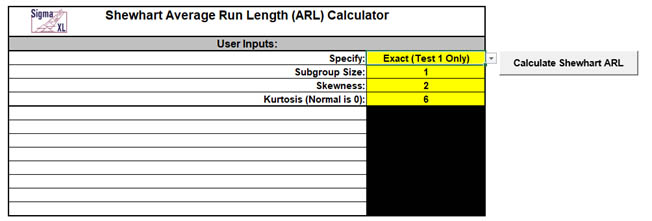Note: We are specifying a severe degree of Skewness (Skewness = 0.5 is mild, 1 is moderate, 2 is severe, and > 2 is very severe). The Pearson family is used to create a distribution that matches the specified Skewness and Kurtosis. Skewness = 2 and Kurtosis = 6 corresponds to an Exponential distribution or Gamma distribution with Shape= 1 and Scale= 1 (for Gamma, Skewness = 2/√Shape and Kurtosis = 6/Shape).

7. Click the Calculate Shewhart ARL button to produce the ARL table and chart for these settings: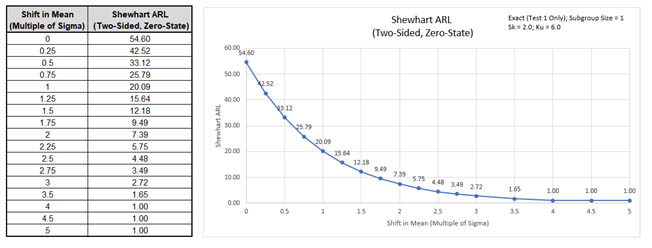ARL0 with these settings is 54.6. This is a very poor performance with a 6.8 x increase (370.4/54.6) in false alarms compared to normal data. The Shewhart Individuals chart is not robust to severe skewness. A Box-Cox Transformation or other Individuals Nonnormal chart should be used (see SigmaXL > Control Charts > Nonnormal > Individuals Nonnormal).

Note: ARL0= 54.6 matches the result given in Montgomery , Table 9.12 for Gam(1,1).

8. Next, we will assess robustness to nonnormality for a Shewhart X-bar chart. Enter Specify = Exact (Test 1 Only), Subgroup Size = 4, Skewness = 2, Kurtosis (Normal is 0) = 6.Note: Skewness of averages = Skewness/√n. Kurtosis of averages = Kurtosis/n. For a subgroup size of 4, the skewness of averages is 1, so is reduced from severe to moderate. Kurtosis of averages is 1.5 (corresponding to a Gamma distribution with Shape = 4).

9. Click the Calculate Shewhart ARL button to produce the ARL table and chart for these settings:ARL0 with these settings is 96.75. This is an improvement over the Individuals 54.6, but is still a 3.8 x increase (370.4/96.75) in false alarms compared to normal data.

Note: ARL0 = 96.75 matches the results given in Schilling & Nelson  (Table 1, Gamma, shape = 1, n=4), =1/.01034. In Table 2, they point out that a subgroup size of 166 would be required to achieve robustness for this severe skewness.

10. Now we will use Monte Carlo simulation to obtain approximate Run Length standard deviation and percentiles for an Individuals Shewhart chart with normal data. Enter Specify = Monte Carlo, Subgroup Size = 1, Skewness = 0, Kurtosis (Normal is 0) = 0, Number of Replications = 1e4, and Test 2 to Test 8 = N/A.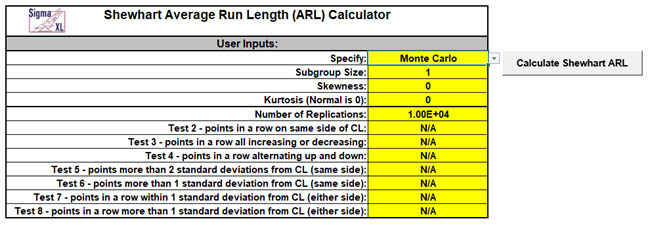11. Click the Calculate Shewhart ARL button to produce the Monte Carlo approximate ARL table, ARL chart and Run Length Standard Deviation and Percentiles table (scroll right to view). Monte Carlo simulation with 10,000 (1e4) replications will take about a minute to run.The additional run length statistics show the large variation of run length values. The median MRL0 = 257 (in-control median run length with 0 shift in process mean). The run length percentiles approximately match those given in Chakraborti  (Table 1, Standards Known, Shift 0.0).

Note: The results will vary slightly since this is Monte Carlo simulation.

12. Now we will use Monte Carlo simulation to assess the Shewhart Individuals chart with all 8 Tests for Special Causes applied. Enter Specify = Monte Carlo, Subgroup Size = 1, Skewness = 0, Kurtosis (Normal is 0) = 0, Number of Replications = 1e4, Test 2 = 9, Test 3 = 6, Test 4 = 14, Test 5 = 2 out of 3, Test 6 = 4 out of 5, Test 7 = 15 and Test 8 = 8.Note: These are the test settings used as defaults in SigmaXL > Control Charts > ‘Tests for Special Causes’ Defaults. Test 1 is always applied.

13. Click the Calculate Shewhart ARL button to produce the Monte Carlo approximate ARL table, ARL chart and Run Length Standard Deviation and Percentiles table.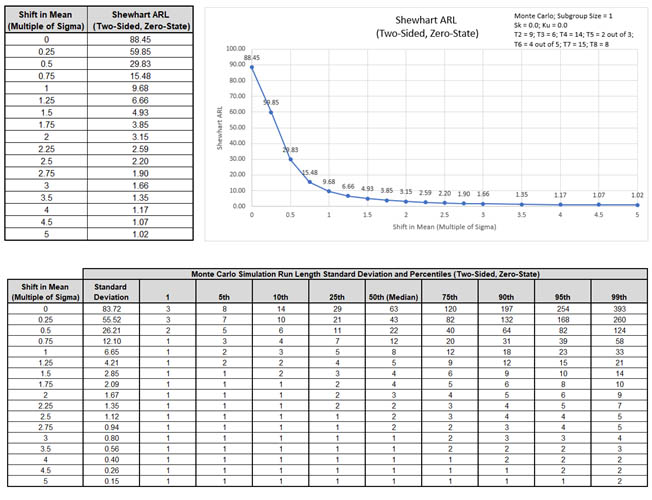ARL0 with all 8 tests for special causes is approx. 88.5. This is a poor performance with a 4.2 x increase (370.4/88.5) in false alarms compared to Test 1 only. MRL0 is approx. 63. On the other hand, ARL1 for a small 1 sigma shift in mean is approx. 9.7, so is much faster to detect than the Exact Test 1 only ARL1 of 43.89.

If small shifts are to be detected quickly and subgrouping is not possible, then an EWMA or CUSUM chart is recommended.

# Template Notes:

1. Specify Exact (Test 1 Only) or Monte Carlo using the drop-down list. Parameters to be specified will be shown in yellow highlight, otherwise they are hidden.
2. Exact uses the cumulative distribution function and is fast. Monte Carlo simulation allows you to assess the ARL performance of all 8 Tests for Special Causes and also produces the table of Run Length Standard Deviation and Percentiles (scroll right to view). Both methods allow you to assess robustness to nonnormality.
3. Test 1 - 1 point more than 3 standard deviations from the center line (CL) is always applied.
4. The Pearson Family of distributions is used to match the specified Skewness and Kurtosis.
5. Enter the Subgroup Size. Subgroup size = 1 denotes a Shewhart Individuals chart. Subgroup size > 1 is an X-Bar chart.
6. Enter Skewness. Skewness = 0 is symmetric.
7. Enter Kurtosis (Normal is 0). Also known as Excess Kurtosis, it must be >= Skewness^2 - 1.48. This is required to keep the distribution unimodal. If Skewness=0 and Kurtosis = 0, the distribution is normal.
8. If applicable, enter Number of Replications. 1000 (1e3) replications will be fast, approx. 10 seconds, but will have an ARL0 error approx. = +/- 10%; 10,000 (1e4) replications will take about a minute, with an ARL0 error = +/- 3.2%; 100,000 (1e5) replications will take about ten minutes, with an ARL0 error = +/- 1%.
9. If applicable, select values for Tests 2 to 8 using the drop-down list. "N/A" indicates that the test is not applied. Tests 2, 3 and 7 provide options that match those provided in SigmaXL's 'Tests for Special Causes' Defaults dialog.
10. Click the Calculate Shewhart ARL button to produce the ARL table and chart. If Monte Carlo was selected, the table of Run Length Standard Deviation and Percentiles will also be produced.
11. The Shewhart ARL is for a two-sided chart with zero-state, i.e., the shift is assumed to occur at the start. The mean and standard deviation are also assumed to be known. This will not likely be the case in use, but is still useful for determining parameter settings and comparison of ARL across chart types.
12. Due to the complexity of calculations, SigmaXL must be loaded and appear on the menu in order for this template to function. Do not add or delete rows or columns in this template.

# REFERENCES:

1. Champ, C.W. and Woodall, W.H. (1987), "Exact results for Shewhart control charts with supplementary runs rules", Technometrics 29, 393-399.
2. Montgomery, D.C. (2013), Introduction to Statistical Quality Control, Seventh Ed., Wiley.
3. Schilling, E. G., and P. R. Nelson (1976), “The Effect of Nonnormality on the Control Limits of X ̅ Charts,”Journal of Quality Technology, Vol. 8(4), pp. 183–188.
4. Chakraborti, S. (2007), “Run Length Distribution and Percentiles: The Shewhart Chart with Unknown Parameters”, Quality Engineering 19, 119–127.

# Web Demos

Our CTO and Co-Founder, John Noguera, regularly hosts free Web Demos featuring SigmaXL and DiscoverSim